# Methods and formulas for Laney P' Chart

Select the method or formula of your choice.

## Plotted points

Each plotted point represents the proportion of defectives for one subgroup, pi:

Data points on a P chart follow the binomial distribution.

### Notation

TermDescription
xinumber of defectives for subgroup i
nisize of subgroup i

## Center line

The center line represents the proportion of defectives for your process,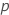. If you do not specify a historical value, then Minitab uses the average proportion of defectives from your data,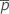, to estimate.

### Notation

TermDescription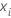number of defectives in subgroup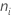size of subgroup## Sigma Z

Each subgroup proportion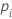is converted to a z-score (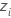):

Next, a moving range of length 2 is used to evaluate the variation in the z-scores and calculate Sigma Z (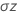):

where 1.128 is an unbiasing constant.

### Notation

TermDescriptionproportion of defectives for subgroupprocess proportionsize of subgroup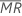moving range of length 2

## Control limits

### Lower control limit (LCL)

The LCL for each subgroupis equal to the greater of the following:

or

### Upper control limit (UCL)

The UCL for each subgroupis equal to the lesser of the following:

or

### Notation

TermDescriptionprocess proportion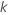parameter for Test 1. The default is 3. (For more information, go to Select tests for special causes for Laney P' Chart.)size of subgroupsee "Sigma Z"